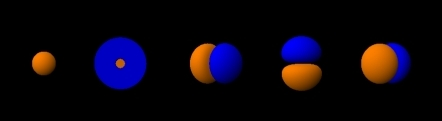# Electron cloud facts for kids

Kids Encyclopedia FactsThe shapes of the first five atomic orbitals are: 1s, 2s, 2px, 2py, and 2pz. The two colors show the phase or sign of the wave function in each region. These are graphs of ψ(x, y, z) functions which depend on the coordinates of one electron. To see the elongated shape of ψ(x, y, z)2 functions that show probability density more directly, see the graphs of d-orbitals below.

Electron cloud is an informal term in physics. It is used to describe where electrons are when they go around the nucleus of an atom.

The electron cloud model is different from the older Bohr atomic model by Niels Bohr. Bohr talked about electrons orbiting the nucleus. Explaining the behavior of these electron "orbits" was a key issue in the development of quantum mechanics.

The electron cloud model says that we can not know exactly where an electron is at any given time, but the electrons are more likely to be in specific areas. It is theoretically possible, for an electron to be a nearly infinite distance away from the atomic nucleus it is orbiting, although the probability of an electron decreases dramatically the further away from the nucleus you search. This is the most modern and accepted way to describe the situation.

In the Bohr model, electrons were assigned to different shells. These shells explained the repeating patterns of chemical properties in the periodic table. Using quantum mechanics, chemists can use the electron cloud model to assign electrons to different atomic orbitals. These atomic orbitals are not all spheres. Atomic orbitals also explain the patterns in the periodic table.

The electron cloud model was developed in 1925 by Erwin Schrödinger and Werner Heisenberg. The model is a way to help visualize the most probable position of electrons in an atom. The electron cloud model is the current accepted model of an atom.

## Images for kidsElectron cloud Facts for Kids. Kiddle Encyclopedia.